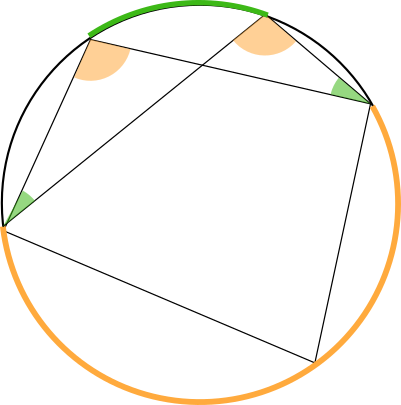# What's that thing about arcs and angles?

• Module 2 Week 2 Day 8 Challenge Explanation Part 1

Why are so many of these challenges about circles? Is there any way I can do these problems without circles because I find them very indefinite and confusing.

• @TSS-Graviser Circles are nice! Of course, if it's the first time you are seeing these types of questions, they will seem inscrutable and incomprehensible. However, that means this is really worth learning. Remember the phrase "from zero to 60 mph in __ seconds," usually conjured in reference to race cars? Well, when you learn something totally new, your logical abilities go "from zero to 60 mph."

Over time, there are some neat patterns you can notice with circles:

• If you connect an exterior point with tangent lines to the circle, the lines are symmetric, and their lengths are the same
• If you see angles inscribed in circles, always look for which arcs they are opened up to. Are there any other angles that open up to the same arc? If so, then they are the same size, no matter how far apart they seem to be!
• If you have a right angle inscribed in a circle, it always opens up to half of the circle, which means the right triangle that you can make from it has a hypotenuse that is really the diameter of the circle!
• If you have any sort of right triangle, the corner of the right angle sits on a circle that you can draw by using the hypotenuse as a diameter

These circle questions show you things that you never could have dreamed would be true without the help of mathematics!

• What's that thing about arcs and all that?

• And what's all the fuss about angles?

• @TSS-Graviser The arcs and the angles actually go together.An arc is a fragment of the line of a circle. In the picture below, the yellow angle inscribes the yellow arc, because you can see that the ends of the angle's segments meet the ends of the yellow arc.Now, I've drawn another angle that also inscribes the yellow arc. This angle is equal to the first yellow angle!Isn't that neat? This is also how we are able to prove the Power of a Point Theorem. Do you see that since the yellow angles are the same, we can form similar triangles? It's because ---- look! There are two more angles that inscribe the same arc!The green angles inscribe the same green arc, and so they are the same as well!

Don't worry if it takes a few times of seeing these ideas before they sink in. It's natural when you are learning something unfamiliar. Remember the saying, "You have to spend 10,000 hours doing anything in order to become an expert." So don't worry if takes time, because this is part of the process of attaining real understanding!